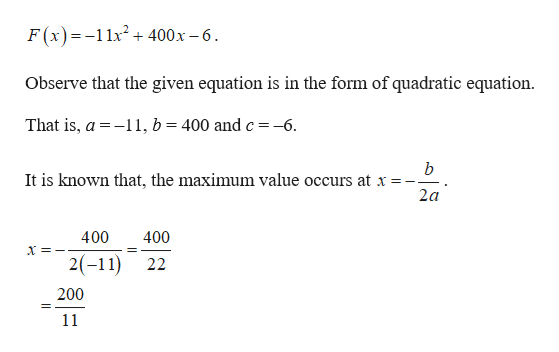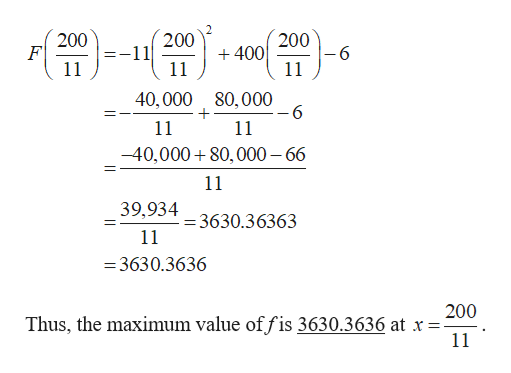# Let F(X)=400x-11x2-6. Find the maximum value of f to four decimal places graphically.The maximum value of f is?

Question
63 views

Let F(X)=400x-11x2-6. Find the maximum value of f to four decimal places graphically.

The maximum value of f is?

check_circle

Step 1

The given function is,help_outlineImage TranscriptioncloseF (x) =-11x + 400x – 6. Observe that the given equation is in the form of quadratic equation. That is, a = -11, b = 400 and c=-6. It is known that, the maximum value occurs at x =- 2a 400 400 X =-- 2(-11) 22 200 11 fullscreen
Step 2

In order to find the maximum value, substitute the...help_outlineImage Transcriptionclose200 F 11 200 -11 200 + 400 -6 11 40, 000 80,000 -6 11 11 -40,000 + 80,000 – 66 11 39,934 = 3630.36363 11 = 3630.3636 200 Thus, the maximum value offis 3630.3636 at x =- 11 fullscreen

### Want to see the full answer?

See Solution

#### Want to see this answer and more?

Solutions are written by subject experts who are available 24/7. Questions are typically answered within 1 hour.*

See Solution
*Response times may vary by subject and question.
Tagged in
MathAlgebra

### Other